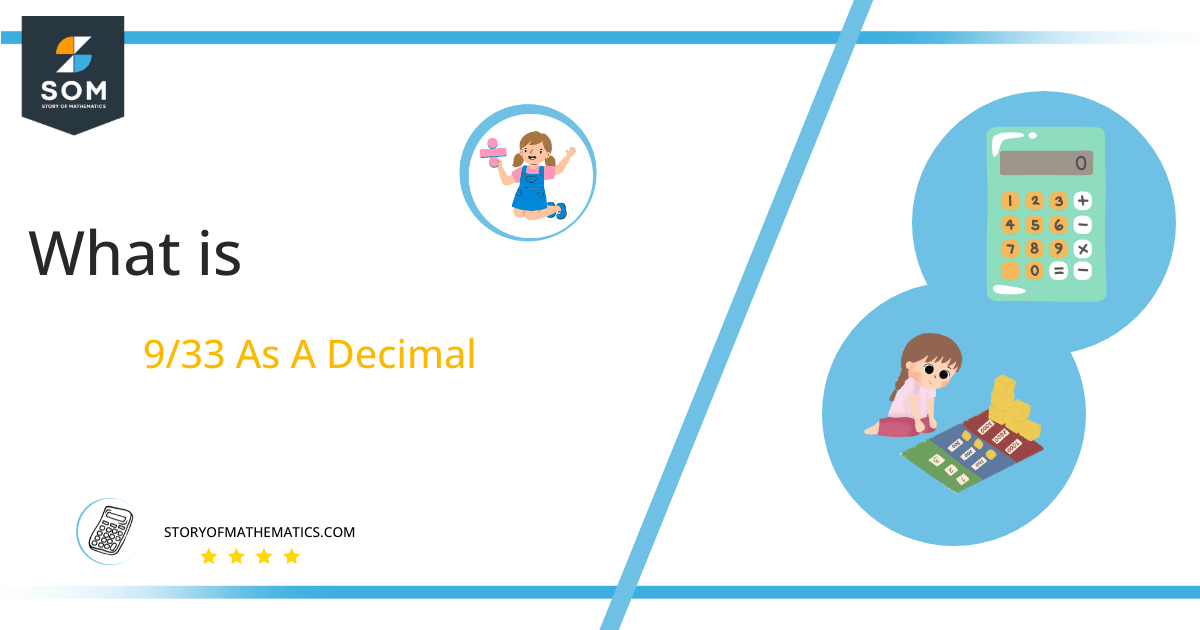# What Is 9/33 as a Decimal + Solution With Free Steps

The fraction 9/33 as a decimal is equal to 0.272.

The fraction 9/33 is a non-terminating decimal fraction as it gives us infinite terms after applying the division method. As a result, we will get decimal notation which is an alternative method to represent the fractional value.

Here, we are more interested in the division types that result in a Decimal value, as this can be expressed as a Fraction. We see fractions as a way of showing two numbers having the operation of Division between them that result in a value that lies between two Integers.Now, we introduce the method used to solve said fraction to decimal conversion, called Long Division, which we will discuss in detail moving forward. So, let’s go through the Solution of fraction 9/33.

## Solution

First, we convert the fraction components, i.e., the numerator and the denominator, and transform them into the division constituents, i.e., the Dividend and the Divisor, respectively.

This can be done as follows:

Dividend = 9

Divisor = 33

Now, we introduce the most important quantity in our division process: the Quotient. The value represents the Solution to our division and can be expressed as having the following relationship with the Division constituents:

Quotient = Dividend $\div$ Divisor = 9 $\div$ 33

This is when we go through the Long Division solution to our problem. The following figure shows the solution for fraction 9/33.Figure 1

## 9/33 Long Division Method

We start solving a problem using the Long Division Method by first taking apart the division’s components and comparing them. As we have 9 and 33, we can see how 9 is Smaller than 33, and to solve this division, we require that 9 be Bigger than 33.

This is done by multiplying the dividend by 10 and checking whether it is bigger than the divisor or not. If so, we calculate the Multiple of the divisor closest to the dividend and subtract it from the Dividend. This produces the Remainder, which we then use as the dividend later.

Now, we begin solving for our dividend 9, which after getting multiplied by 10 becomes 90.

We take this 90 and divide it by 33; this can be done as follows:

90 $\div$ 33 $\approx$ 2

Where:

33 x 2 = 66

This will lead to the generation of a Remainder equal to 90 – 66 = 24. Now this means we have to repeat the process by Converting the 24 into 240 and solving for that:

240 $\div$ 33 $\approx$ 7

Where:

33 x 7 = 231

This, therefore, produces another Remainder which is equal to 240 – 231 = 9. Now this means we have to repeat the process by Converting the 9 into 90 and solving for that:

90 $\div$ 33 $\approx$ 2

Where:

33 x 2 = 66

Finally, we have a Quotient generated after combining the three pieces of it as 0.272, with a Remainder equal to 24.Images/mathematical drawings are created with GeoGebra.